# Bonus

Gross wage was 527 EUR including 16% bonus.

How many EUR were bonuses?

Result

b =  72.69 EUR

#### Solution:

$\ \\ a + 16/100 \ a=527 \ \\ \ \\ 116a=52700 \ \\ \ \\ a=\dfrac{ 13175 }{ 29 } \doteq 454.310345 \ \\ b=527 - a \doteq 527 - 454.3103 \doteq \dfrac{ 2108 }{ 29 } \doteq 72.6897 \ \\ \ \\ \ \\ t=a+b=454.3103+72.6897=527 \ \\ b=q=100 \cdot \ \dfrac{ b }{ t-b }=100 \cdot \ \dfrac{ 72.6897 }{ 527-72.6897 }=16 \ \%=72.69 \ \text{EUR}$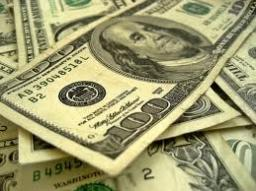Our examples were largely sent or created by pupils and students themselves. Therefore, we would be pleased if you could send us any errors you found, spelling mistakes, or rephasing the example. Thank you!

Leave us a comment of this math problem and its solution (i.e. if it is still somewhat unclear...):Be the first to comment!Tips to related online calculators
Need help calculate sum, simplify or multiply fractions? Try our fraction calculator.
Check out our ratio calculator.
Do you have a linear equation or system of equations and looking for its solution? Or do you have quadratic equation?

## Next similar math problems:

1. Corona again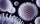If the population on long island is 7.75 million and the coronavirus has affected 22,178. What percent of the population is affected. (looking for 1 in? If possible)
2. ChestnutsThree divisions of nature protectors participated in the collection of chestnut trees.1. the division harvested 1250 kg, the 2nd division by a fifth more than the 1st division and the 3rd division by a sixth more than the second division. How many tons of
3. The fourThe four pirates divided 65 coins to each other. They were sorted by age, the youngest receiving the least number of coins, each half more than the previous one. How many coins did the oldest pirate receive?
4. LibraryNew books were purchased for the library. Five-eighths were professional books, one-fifth were encyclopedias, and 231 books were dictionaries. How many professional books were there?
5. Triangular prismThe base of the perpendicular triangular prism is a rectangular triangle with a hypotenuse of 10 cm and one leg of 8 cm. The prism height is 75% of the perimeter of the base. Calculate the volume and surface of the prism.Adam has half the money in his right pocket than in his left pocket. If he transferred 40 crowns from the left pocket to the right, he would have the same in both pockets. Calculate how many crowns does Adam have in his left pocket more than in his right?
7. Job applicantsJob applicants: three-fourths of applicants had experience for a position. The number that did not have prior experience was 36. How many people applied for the job?
8. Cllub flag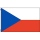The children had the club flag sewn on. The red color covered 2/20 of the total area of the flag, the blue area a fifth, the yellow 19% of the total area. What% is the green area occupied?
9. Half of halvesHalf of the square we cut off, then half of the rest, etc. Five cuts we made in this way. What part of the content of the original square is the content of the cut part?
10. Interior anglesCalculate the interior angles of a triangle that are in the ratio 2: 3: 4.
11. The angles ratioThe angles in the ABC triangle are in the ratio 1: 2: 3. find the sizes of the angles and determine what kind of a triangle it is.
12. A bucketA bucket has 4 liters of water in it when it is 2/5 full. How much can it hold?
13. The angles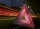The angles in the triangle are in the ratio 12: 15: 9. Find the angles.
14. Brownies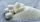Mrs. Merritt made brownies, the recipe had to be cut in 1/2. The recipe called for 2 5/8 cups of sugar. How much sugar did she use?
15. Lookout towerCalculate the height of a lookout tower forming a shadow of 36 m if at the same time a column 2.5 m high has a shadow of 1.5 m.
16. Garden exchangeThe garden has the shape of a rectangular trapezoid, the bases of which have dimensions of 60 m and 30 m and a vertical arm of 40 m. The owner exchanged this garden for a parallelogram, the area of which is 7/9 of the area of a trapezoidal garden. What is
17. Simplest form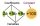Find the simplest form of the following expression: 3 to the 2nd power - 1/4 to the 2nd power